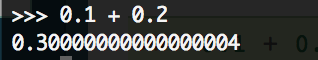### Float like a butterfly; sting like a bee.

Your hands can't hit what your eyes can't see.

For this reason, we have to be careful when feeding numbers into our computer. Check out what happens when you ask a basic math question to Python:Pictured: the folly of man

Huh, that's strange. Where did all those zeros come from? It turns out that machines use binary to represent integers. This means that, for the number .13, instead of summing 0/1 + 1/10 + 3/100,  the computer must use 0/2 + 0/4 + 1/8 + ... whatever else comes after that in binary code. (Python calls this a float.) Make sure you keep this in mind when working with mathematically intensive projects.

Pi is an oddity. It's never-ending just like our efforts to calculate it. At the same time, it's always nice to appreciate how there are so many different ways to calculate pi. For example, you could drop needles. And, sure, we can always measure the ratio of the circumference of a circle to its diameter, but how do we tell a computer, a fundamentally deterministic and causal entity, how to calculate pi? Let's ask two different programming languages and see what they have to say.

Python:
pi=4*np.arctan2(1,1)

Fortran:
double precision pi
pi=4.d0*datan(1.d0)

(Notice the "d" in the Fortran statement. That tells us the double precision, or, to what power of ten we multiple our number.)

It appears Python and Fortran are in consensus about this one. If you use a bit of intuition when reading the two statements, you might be able to tell that they are both defining pi as four times the angle created from arctan 1. But what value of pi do they both actually give us?

Python: 3.1415926535897931
Fortran: 3.1415926535897931

According to Value of Pi, pi is 3.1415926535897932384...with several different computational techniques. Looks like it's a tie for this round.

I've found that working with two different languages on the same project forces you to really understand the syntax and meaning behind each of the languages. With my example of Fortran and Python, We can really see the difference between the two.